Skip to main content
$$\require{cancel}$$

# 13.9: Chapter 9

Problem (9.1).

A plane wave is polarized with its electric vector along z. The wave propagates along the y-axis. The electric field is given by

$\begin{equation}\text{E}_{2}(\text{y}, \text{t})=\text{E}_{0} \text{e}^{\text{i}(\text{ky}-\omega \text{t})} \quad \text { Volts/meter. }\end{equation}\nonumber$

This wave is propagating in vacuum; its amplitude is Eo = 5V/m and its wavelength is 0.10 meters.

1. What is the frequency of the wave?
2. How large is the magnetic field associated with this wave and in what direction is it oriented?
3. What is the average rate at which energy is transported by this wave (per square meter)?
4. This wave encounters an electron. At what rate does the electron remove energy from the wave?
5. The wave propagates through an electron gas whose density is 1015 per cubic centimeter. If each electron acts like an independent scattering center estimate the distance the wave will travel before its amplitude has been reduced to (1/e) of its initial value.

Answer (9.1).

a) ω = ck in free space

$$\therefore f=\frac{c}{\lambda}=3 \times 10^{9} \text{Hz}=3 \text{GHz}$$

b) B is along x:$$\begin{equation}\text{B}_{\text{x}}=\frac{\text{E}_{\text{O}}}{\text{c}} \text{e}^{\text{i}(\text{k} \text{y}-\omega \text{t})}\end{equation}$$

$$\therefore \text{H}_{\text{X}}=\frac{\text{E}_{\text{O}}}{\text{c} \mu_{\text{O}}} e^{\text{i}(\text{ky}-\omega \text{t})}=\text{H}_{\text{O}} \text{e}^{\text{i}(\text{ky}-\omega t)}$$

$$\begin{equation}\therefore \text{H}_{0}=\frac{\text{E}_{0}}{\text{c} \mu_{0}}=\frac{5}{\left(3 \times 10^{8}\right)\left(4 \pi \times 10^{-7}\right)}=\frac{5}{120 \pi}=\frac{5}{377}\end{equation}$$

$$\text{H}_{0}=13.26 \times 10^{-3} \mathbf{Amps/meter}$$.

(c) $$S_{Y}=E_{z} H_{X}=(5)\left(13.3 \times 10^{-3}\right) \cos ^{2}(k y-\omega t)$$

$$\left\langle S_{Y}\right\rangle=\left(\frac{1}{2}\right)(5)(13.3) \times 10^{-3} \text {Watts } / m^{2}=\mathbf{33.16 \times 10^{-3}}$$ Watts/m2.

d) For the electron $$\text{ma}=-|e| \text{E}_{0} \text{e}^{-\text{i} \omega \text{t}}$$

$\therefore a=-\frac{|e|}{m} E_{0} e^{-i \omega t} \nonumber$

The energy scattered by an accelerated charge and integrated over all angles is given by

$$\frac{d W}{d t}=\left(\frac{2}{3}\right) \left(\frac{1}{4 \pi \varepsilon_{0}}\right) \frac{|e|^{2}}{c^{3}} a^{2}$$

Time Average: $$\left\langle\frac{d W}{d t}\right\rangle=\left(\frac{1}{3}\right) \left(\frac{1}{4 \pi \varepsilon_{0}}\right) \frac{e^{4}}{m^{2} c^{3}} E_{0}^{2}$$

or $$\left\langle\frac{d W}{d t}\right\rangle=0.88 \times 10^{-31} E_{0}^{2}$$ since $$\frac{e^{4}}{m^{2} c^{4}}=9.80 \times 10^{-50}$$.

Eo = 5 V/m initially so in this case

$\mathbf{\left\langle\frac{d W}{d t}\right\rangle=22.05 \times 10^{-31} \text {Watts. }}\nonumber$

e) Now $$\frac{d W}{d t}=\alpha E_{0}^{2}$$ for 1 electron.

There are 1015 electrons/cc = 1021 electrons/m3 = N. Consider a section of the wave having an area of 1 m2 and look at the energy change in traversing a distance dy:

The energy change in dy is

$\Delta \text{W}=-\left(\alpha \text{E}^{2}\right)(\text{N} \text{dy})=-\text{N} \alpha \text{dy} \text{E}^{2}\nonumber$

But the Poynting vector is given by (time average)

$$<S>=\frac{E^{2}}{2 c \mu}=\frac{E^{2}}{240 \pi}$$

$$\therefore \quad<S>\quad(y+d y)-\langle S(y)\rangle=-N \alpha E^{2} \quad d y$$

or $$\text{d} \text{y} \frac{<\text{d} S>}{\text{d} \text{y}}=-\text{N} \alpha \text{E}^{2} \quad \text{d} \text{y}$$

or $$\frac{<d S>}{d y}=-N \alpha E^{2}$$.

But $$\langle S\rangle=\frac{E^{2}}{240 \pi}$$

$$\frac{d<S>}{d y}=\frac{E}{120 \pi} \frac{d E}{d y}$$

$$\therefore \frac{E}{120 \pi} \frac{d E}{d y}=-N \alpha E^{2}$$

or $$\frac{d E}{d y}=-N \alpha(120 \pi) E=-377 \text{N} \alpha E$$

where $$\alpha=0.88 \times 10^{-31} \frac{\text { Watts }}{V^{2}}$$ and $$\text{N}=10^{21} \text { electrons } / \text{m}^{3}$$.

$$\therefore \text{E}=\text{E}_{\text{O}} \text{e}^{-\text{y} / \text{L}}$$

where $$1 / L=(377)(N) \quad \alpha=0.33 \times 10^{-7} \text{m}^{-1}$$

or L = 3.01 x 107 meters = 3.01 x 104 km.

So the wave can travel ~ 30,000 km before its amplitude has dropped to e-1 of its initial value.

Problem (9.2).

A plane wave is propagating thru empty space with a wavevector given by

$\mathbf{k}=6.283 \frac{\left(\hat{\mathbf{u}}_{\text{x}}+\hat{\mathbf{u}}_{\text{y}}\right)}{\sqrt{2}} \quad \text { per meter. }\nonumber$

The electric vector has a strength of $$\frac{1}{10}$$ Volts/meter.

1. Calculate the frequency and wavelength of this radiation.
2. How large is the magnetic field B associated with this wave.
3. At what average rate is energy being transported by this wave (Watts/meter2).
4. What is the average stored electrical energy in the wave? (Joules/m3)
5. What is the average stored magnetic energy in the wave? (Joules/m3).

Answer (9.2).

Assume that the wave is polarized with E along z - this will ensure that k·E = 0. Then

$\text{E}_{\text{z}}=\text{E}_{\text{O}} \text{e}^{\text{i}\left(\text{k}_{\text{x}} \text{x}+\text{k}_{\text{y}} \text{y}\right)} \cdot \text{e}^{-\text{i} \omega \text{t}}\nonumber$

where $$k_{x}=\frac{2 \pi}{\sqrt{2}}$$ and $$k_{Y}=\frac{2 \pi}{\sqrt{2}}$$ meters-1.

But $$\operatorname{curl} \mathbf{E}=-\frac{\partial \mathbf{B}}{\partial \text{t}}=\text{i} \omega \mathbf{B}$$

$$\operatorname{curl} \mathbf{E}=\left|\begin{array}{ccc} \hat{\mathbf{u}}_{\text{x}} & \hat{\mathbf{u}}_{\text{y}} & \hat{\mathbf{u}}_{\text{z}} \\ \frac{\partial}{\partial \text{x}} & \frac{\partial}{\partial \text{y}} & \frac{\partial}{\partial \text{z}} \\ 0 & 0 & \text{E}_{\text{z}} \end{array}\right|=\left|\begin{array}{c} \frac{\partial \text{E}_{\text{z}}}{\partial \text{y}} \\ -\frac{\partial \text{E}_{\text{z}}}{\partial \text{x}} \\ 0 \end{array}\right|$$

$$\therefore i \omega B_{x}=i k_{y} E_{z}$$ or $$\text{B}_{\text{X}}=\left(\frac{\text{k}_{\text{Y}}}{\omega}\right) \text{E}_{\text{Z}}$$

$$\text{i} \omega \text{B}_{\text{y}}=-\text{i} \text{k}_{\text{X}} \text{E}_{\text{z}}$$ $$\text{B}_{\text{Y}}=-\left(\frac{\text{k}_{\text{X}}}{\omega}\right) \quad \text{E}_{\text{z}}$$

Now $$\frac{\omega}{c}=2 \pi$$ ∴ f = 3 x 108 Hz i.e. 300 MHz

and $$\lambda$$ = 1 meter.

$$\text{H}_{\text{x}}=\frac{\text{B}_{\text{X}}}{\text{\mu}_{\text{O}}}=\frac{\text{E}_{\text{O}}}{\text{C} \mu_{\text{O}} \sqrt{2}} \text{e}^{\text{i}\left(\text{k}_{\text{X}} \text{x}+\text{k}_{\text{Y}} \text{Y}-\omega \text{t}\right)}$$

$$\text{H}_{\text{Y}}=\frac{\text{B}_{\text{Y}}}{\mu_{\text{O}}}=-\frac{\text{E}_{\text{O}}}{\text{C} \mu_{\text{O}} \sqrt{2}} e^{\text{i}\left(\text{k}_{\text{X}} \text{x}+\text{k}_{\text{Y}} \text{Y}-\omega \text{t}\right)}$$

where cµo = (3 x 108)(4$$\pi$$ x 10-7) = 120 $$\pi$$ = 377 Ohms. The Poynting Vector is S = E x H and it is directed along k. Since E and H are perpendicular

$|\mathbf{E} \times \mathbf{H}|=\frac{E_{0}^{2}}{C \mu_{0}} \cos ^{2}\left(k_{X} \mathbf{x}+k_{Y Y}-\omega t\right)\nonumber$

Time Average $$<S>=\frac{E_{0}^{2}}{2 c \mu_{0}}=\frac{.01}{(2)(377)}=\mathbf{1.326 \times 10^{-5} \text {Watts } / \mathbf{m}^{2}}$$

Amplitude $$|\mathbf{B}|=\sqrt{B_{x}^{2}+B_{y}^{2}}=\frac{E_{0}}{C}=\frac{10^{-1}}{3 \times 10^{8}}=\mathbf{3.333 \times 10^{-10} \mathbf{Tesla}}$$

Amplitude $$|\mathbf{H}|=\frac{|\mathbf{B}|}{\mu_{0}}=\frac{10^{-1}}{377}=\mathbf{2.652 \times 10^{-4} \mathbf{Amps } / \mathbf{m}}$$.

(d) Energy density stored in the electric field is given by

$w_{E}=\frac{\varepsilon_{0} E^{2}}{2}\nonumber$

$\text{w}_{\text{E}}=\frac{\varepsilon_{\text{o}} \text{E}_{\text{O}}^{2}}{2} \cos ^{2}\left(\text{k}_{\text{x}} \text{x}+\text{k}_{\text{y} \text{Y}}-\omega \text{t}\right)\nonumber$

$\therefore \operatorname{Avg}<w_{E}>=\frac{\varepsilon_{0} E_{0}^{2}}{4}=\frac{E_{0}^{2}}{(4)(36 \pi)\left(10^{9}\right)}\nonumber$

$\mathbf{=2.21 \times 10^{-14} \text {Joules } / \text{m}^{3}}.\nonumber$

(e) The average energy stored in the magnetic field is given by

$<w_{B}>=\frac{\left|B_{0}\right|^{2}}{4 \mu_{0}}=\frac{\varepsilon_{0}}{4} E_{0}^{2}=\mathbf{2.21 \times 10^{-14} \text {Joules } / \mathbf{m}^{3}}\nonumber$

Problem (9.3).

The electric field of an electromagnetic wave

$\mathbf{E}=\text{E}_{0} \hat{\mathbf{u}}_{\text{x}} \cos \left[10^{8} \pi\left(\text{t}-\frac{\text{z}}{\text{c}}\right)+\theta\right] \quad \text{V} / \text{m}\nonumber$

is the sum of

$\mathbf{E}_{1}=0.03 \hat{\mathbf{u}}_{\text{x}} \sin 10^{8} \pi\left(\text{t}-\frac{\text{z}}{\text{c}}\right) \quad \text{V} / \text{m}\nonumber$

and $$\mathbf{E}_{2}=0.04 \hat{\mathbf{u}}_{x} \cos \left[10^{8} \pi\left(t-\frac{z}{c}\right)-\frac{\pi}{3}\right] \quad V / m$$

Find Eo and θ.

Answer (9.3).

The electric field can be written

$$E_{x}=E_{0} \cos \theta \cos 10^{8} \pi\left(t-\frac{z}{c}\right)-E_{0} \sin \theta \sin 10^{8} \pi\left(t-\frac{z}{c}\right)$$

Also $$\text{E}_{1 \text{x}}=0.03\left[\sin 10^{8} \pi\left(\text{t}-\frac{\text{z}}{\text{c}}\right)\right]$$

and $$E_{2 x}=0.04\left[\cos \left(\frac{\pi}{3}\right) \cos 10^{8} \pi\left(t-\frac{z}{c}\right)\right.+\sin \left(\frac{\pi}{3}\right) \sin 10^{8} \pi\left(t-\frac{z}{c}\right)$$

$$\therefore E_{1 x}+E_{2 x}=0.02 \cos 10^{8} \pi\left(t-\frac{z}{c}\right)+0.0646 \sin 10^{8} \pi\left(t-\frac{z}{c}\right)$$

∴ compare with above. Eo cos θ = 0.02

-Eo sin θ = 0.06464

$$\therefore \tan \theta=-\frac{0.06464}{0.02}=-3.232$$ $$\therefore \theta=\mathbf{-72.81^{\circ}=-1.271 \pi}$$.

and $$E_{0}=\frac{0.02}{\cos \theta}=\mathbf{0.06766 \quad v / m}$$.

Problem (9.4).

An optical device called a $$\lambda$$/2-plate (half-wave plate) is characterized by two axes which can be labeled x and y. The velocity of a plane wave polarized along y is different from the velocity of a plane wave polarized along x. The plate thickness is such that a phase shift of $$\pi$$ is introduced between waves polarized along x and along y. Consider an incident plane polarized beam of light such that the electric vector makes an angle $$\phi$$ with the x-axis. Show that the plane of polarization of the exit beam will be rotated through 2$$\phi$$. This mechanism is used in experiment to make fine adjustments to the plane of polarization.

(Hint: Decompose the electric field vector of the incident plane wave into the sum of two plane waves; one having the electric vector polarized along x, Ex= E0cos$$\phi$$, the other having the electric vector polarized along y, Ey= E0sin$$\phi$$).

Answer (9.4).This can be written as the sum of two plane waves:

Ex = Eo cos(kz-wt) cos $$\phi$$ = Eo cos$$\phi$$ cosωt (at z=0)

Ey = Eo cos(kz-wt) sin $$\phi$$ = Eo sin$$\phi$$ cosωt (at z=0).

If the y-axis is slow then the exit waves will have the form

$E_{x}=E_{0} \cos \phi \cos \left(k_{x} d-\omega t\right)\nonumber$

$\text{E}_{\text{y}}=\text{E}_{\text{O}} \sin \phi \cos \left(\text{k}_{\text{y}} \text{d}-\omega \text{t}\right).\nonumber$

However, $$\text{v}_{\text{x}}=\frac{\text{c}}{\text{n}_{\text{x}}}$$ and $$\text{V}_{\text{Y}}=\frac{\text{c}}{\text{n}_{\text{Y}}}$$, where nx,ny are the indices of refraction for propagation of light along the x and y axes. One has ω = vxkx and ω = vyky so that if y is a slow axis ky > kx.

therefore Ey = E0 sin$$\phi$$ cos(kxd-ωt+$$\pi$$),

or Ey = - Eo sin$$\phi$$ cos(kxd-ωt).

But Ex = E0cos$$\phi$$ cos(kxd-ωt), so these electric field components correspond to a plane wave in which the direction of polarization has been rotated through 2$$\phi$$ (clockwise). A similar argument also gives 2$$\phi$$ clockwise if x is the slow axis: the phase of the resulting wave is just shifted by 180°.

Problem (9.5).

A quarter wave plate is similar to the half-wave plate of problem (9.4) except that the thickness is adjusted so that in its passage through the plate light polarized parallel with one principle axis is shifted by $$\pi$$/2 in phase relative to light polarized with its electric vector parallel with the other axis. (See the sketch).Let light be incident on the $$\frac{\lambda}{4}$$ - plate which is polarized so that its electric vector makes an angle $$\phi$$ with the fast axis. Show that the transmitted light will be elliptically polarized. For what angle $$\phi$$ will the transmitted light be circularly polarized?

Answer (9.5).

At z = 0 Ex = Eo cos$$\phi$$ cosωt

Ey = Eo sin$$\phi$$ cosωt,

Plane polarized incident light.

At exit where z = d

\begin{aligned} \text{E}_{\text{X}} &=\text{E}_{\text{O}} \cos \phi \cos \left(\text{k}_{\text{X}} \text{d}-\omega \text{t}\right) \\ \text{E}_{\text{Y}} &=\text{E}_{\text{O}} \sin \phi \cos \left(\text{k}_{\text{Y}} \text{d}-\omega \text{t}\right) \\ &=\text{E}_{0} \sin \phi \cos \left(\text{k}_{\text{X}} \text{d}-\omega \text{t}+\frac{\pi}{2}\right) \end{aligned}\nonumber

since y is the slow axis for which ky > kx. This follows from the relations $$\omega=v_{\text{X}} \text{k}_{\text{X}}=\left(\frac{\text{c}}{\text{n}_{\text{X}}}\right) \text{k}_{\text{X}}$$ and $$\omega=\text{v}_{\text{y}} \text{k}_{\text{y}}=\left(\frac{\text{c}}{\text{n}_{\text{Y}}}\right) \text{k}_{\text{Y}}$$, ie. $$k_{Y}=\left(\frac{n_{Y}}{n_{X}}\right)$$.

Thus $$\text{E}_{\text{Y}}=-\text{E}_{\text{O}} \sin \phi \sin \left(\text{k}_{\text{x}} \text{d}-\omega \text{t}\right)$$.

These components can be written

$\begin{array}{l} \text{E}_{\text{X}}=\text{E}_{0} \cos \phi \cos \left(\omega \text{t}-\text{k}_{\text{X}} \text{d}\right) \\ \text{E}_{\text{Y}}=\text{E}_{0} \sin \phi \sin \left(\omega \text{t}-\text{k}_{\text{X}} \text{d}\right) \end{array}. \nonumber$

$$\begin{array}{l|c|l} \left(\omega t-k_{x} d\right) & E_{x} & E_{Y} \\ 0 & \text { E}_0 \cos \phi & 0 \\ \pi / 2 & 0 & +E_{0} \sin \phi \\ \pi / 4 & \frac{E_{0} \cos \phi}{\sqrt{2}} & +\frac{E_{0}}{\sqrt{2}} \sin \phi \\ \pi & -E_{0} \cos \phi & 0 \\ \frac{3 \pi}{2}& 0 & -E_{0} \sin \phi \\ -\pi / 4 & \frac{E_{0} \cos \phi}{\sqrt{2}} & -\frac{E_{0}}{\sqrt{2}} \sin \phi \\ \end{array}$$

The light will become circularly polarized for $$\phi$$= $$\pi$$/4.Problem (9.6).

A charged particle moves in a circular orbit of radius b meters centered on the origin and lying in the x-y plane. The co-ordinates of the particle can be described by the relations

$x = b\cos ωt\nonumber$

$y = b\sin ωt \nonumber$

where ω= 2$$\pi$$f = 3x1015 radians/second. The motion is equivalent to the superposition of two point dipoles

$p_{x}=p_{0} \cos \omega t=Q b \cos \omega t\nonumber$

$\text{p}_{\text{Y}}=\text{p}_{0} \sin \omega \text{t}=\text{Qb} \sin \omega \text{t}\nonumber$

where p0= 10-30 Coulomb-meters.

1. An observer is located at x=0, y=0, z=1 meter. How will the electric field at the observer vary in time? What intensity of radiation will be observed?
2. An observer is located at x=0, y=0.707, z=0.707 meters. How will the electric field at the observer vary in time? What intensity of radiation will be observed?
3. An observer is located at x=0, y=1, z=0 meters. How will the electric field at the observer vary in time? What intensity of radiation will be observed?

Answer (9.6).

(a) The observer is at right angles to both dipoles. The radiation fields are given by (R=1 meter, Sinθ=1)

$\text{E}_{\text{X}}=\frac{1}{4 \pi \varepsilon_{0}}\left(\frac{\omega}{\text{c}}\right)^{2} \text{p}_{0} \cos (\omega \text{t}-\text{R} / \text{c})\nonumber$

$\text{E}_{\text{Y}}=\frac{1}{4 \pi \varepsilon_{0}}\left(\frac{\omega}{\text{c}}\right)^{2} \text{p}_{0} \sin (\omega \text{t}-\text{R} / \text{c})\nonumber$

The electric field is right hand circularly polarized. The intensity of the radiation will just be given by

$S_{z}=\frac{E_{0}^{2}}{Z_{0}}=\frac{1}{Z_{0}}\left(\frac{p_{0}}{4 \pi \varepsilon_{0}}\right)^{2}\left(\frac{\omega}{c}\right)^{4}, \nonumber$

independent of time because $$\cos ^{2}(\omega[t-R / c])+\sin ^{2}(\omega[t-R / c])=1$$. Z0= 377 Ohms, thus Sz = 2.149x10-15 Watts/m2. Notice that the intensity does not fluctuate with time for a circularly polarized wave.

(b) For the observer at (0,0.707,0.707) the electric fields will be given by

$\text{E}_{\text{X}}=\frac{1}{4 \pi \varepsilon_{0}}\left(\frac{\omega}{\text{c}}\right)^{2} \text{p}_{0} \cos (\omega[\text{t}-\text{R} / \text{c}])\nonumber$

$\text{E}_{\theta}=-\frac{1}{4 \pi \varepsilon_{0}}\left(\frac{\omega}{\text{c}}\right)^{2} \text{p}_{0} \frac{\sin (\omega[\text{t}-\text{R} / \text{c}])}{\sqrt{2}}\nonumber$

Therefore

$\text{E}_{\text{Y}}=\frac{1}{4 \pi \varepsilon_{0}}\left(\frac{\omega}{\text{c}}\right)^{2} \text{p}_{0} \frac{\sin (\omega[\text{t}-\text{R} / \text{c}])}{2}\nonumber$

$\text{E}_{\text{Z}}=-\frac{1}{4 \pi \varepsilon_{0}}\left(\frac{\omega}{\text{c}}\right)^{2} \text{p}_{0} \frac{\sin (\omega[\text{t}-\text{R} / \text{c}])}{2}.\nonumber$

This electric field corresponds to right hand elliptically polarized radiation.

In a co-ordinate system rotated so that the new Z-axis is pointed along the line joining the observer to the origin one has

$\text{E}_{\text{X}}=\frac{1}{4 \pi \varepsilon_{0}}\left(\frac{\omega}{\text{c}}\right)^{2} \text{p}_{0} \cos (\omega[\text{t}-\text{R} / \text{c}])\nonumber$

and

$\text{E}_{\eta}=\frac{1}{4 \pi \varepsilon_{0}}\left(\frac{\omega}{\text{c}}\right)^{2} \text{p}_{0} \frac{\sin (\omega[\text{t}-\text{R} / \text{c}])}{\sqrt{2}}.\nonumber$

The time averaged intensity is given by

$<S>=\frac{E_{X}^{2}}{2 Z_{0}}+\frac{E_{\eta}^{2}}{2 Z_{0}},\nonumber$

where Z0= 377 Ohms, and EX,Eη are the electric field amplitudes. In this case $$\text{E}_{\eta}=\text{E}_{\text{X}} / \sqrt{2}$$, so that

$<S>=\left(\frac{3}{4}\right)\left(2.15 \times 10^{-15}\right)=1.611 \times 10^{-15} \text {Watts } / \text{m}^{2}.\nonumber$

(c) An observer at (0,1,0) sees a radiation field due entirely to the dipole oriented along the x-axis. The electric field will be linearly polarized and

$\text{E}_{\text{x}}=\frac{1}{4 \pi \varepsilon_{0}}\left(\frac{\omega}{\text{c}}\right)^{2} \text{p}_{0} \cos (\omega \text{t}-\text{R} / \text{c}). \nonumber$

The corresponding intensity will be just half the intensity measured by the observer on the z-axis:

$\langle S\rangle=1.074 \times 10^{-15} \text {Watts } / \text{m}^{2}.\nonumber$

Problem (9.7).

Consider the sum of 5 phasors:

$S=e^{i \phi}+e^{2 i \phi}+e^{3 i \phi}+e^{4 i \phi}+e^{5 i \phi}.\nonumber$

This is the sum of 5 waves: the phase shift between each pair of waves is $$\phi$$.

1. Calculate the sum for $$\phi$$ = 0
2. Calculate the sum for $$\phi=\frac{\pi}{10}$$
3. Calculate the sum for $$\phi$$ = $$\pi$$/5
4. Calculate the sum for $$\phi=\frac{2 \pi}{5}$$
5. Make a sketch of S as a function of $$\phi$$.

A graphical construction is useful for summing phasors. Notice that one has to do with a geometrical series.

Answer (9.7).

(a) S = 5.0

(b) $$S=e^{i \phi}\left(1+e^{i \phi}+e^{2 i \phi}+e^{3 i \phi}+e^{4 i \phi}\right)$$

$$=e^{i \phi \frac{\left(e^{5 i \phi}-1\right)}{\left(e^{i \phi}-1\right)}}$$

S = 2.657 + 3.657i

|S| = 4.520

Angle = 54.00˚(c) $$\phi=\frac{\pi}{5}=36^{\circ}$$

S = -1.00 + 3.078i

Angle = 108.00˚

|S| = 3.236(d) $$\phi=\frac{2 \pi}{5}=72^{\circ}$$

S = 0(e) (i) When $$\phi$$ is a multiple of 2$$\pi$$ S = 5.0

(ii) The sum is a geometric progression

\begin{aligned} S &=e^{i \phi}\left(1+e^{i \phi}+e^{2 i \phi}+e^{3 i \phi}+e^{4 i \phi}\right) \\ &=e^{i \phi \frac{\left(e^{5 i \phi}-1\right)}{\left(e^{i \phi}-1\right)}} \end{aligned}

$$|S|^{2}=S S^{\star}=\frac{1-\cos 5 \phi}{1-\cos \phi}$$Notice that for N phasors

$|S|^{2}=\frac{1-\cos N \phi}{1-\cos \phi}\nonumber$

So when $$\phi$$ = 0 or 2$$\pi$$ |S|2 = N2 .

These high peaks drop to zero when N$$\phi$$ = 2$$\pi$$ or $$\phi=\frac{2 \pi}{\text{N}}$$ and |S|2 = 0 for multiples of $$\frac{2 \pi}{\text{N}}$$.

There are peaks at $$\phi=(\text { odd integer } \geq 3) \times \frac{\pi}{N}=(g)\left(\frac{\pi}{N}\right)$$.

However $$\cos \left(\frac{g \pi}{N}\right) \simeq 1-\frac{g^{2} \pi^{2}}{2 N^{2}}$$

$$\therefore|\text{S}|^{2} \cong \frac{4 \text{N}^{2}}{\text{g}^{2} \pi^{2}} \sim\left(\frac{.41}{\text{g}^{2}}\right) \text{N}^{2}$$, so these subsidiary peaks drop off as .045, .0162, etc. and |S|2 drops off rapidly with phase angle.Problem (9.8).

Eight atoms are located on the corners of a cube whose sides are a long. One corner of the cube is located at the origin of co-ordinates, and the sides of the cube are parallel with the co-ordinate axes. The polarizability of each atom is $$\alpha$$, i.e. in the presence of an electric field the atom developes a dipole moment given by p= $$\alpha$$E. Let an incident free space plane wave of the form

$\text{E}_{\text{z}}=\text{E}_{0} \text{e}^{\text{i}(\text{kx}-\omega \text{t})}\nonumber$

fall on the group of 8 atoms, where k= 2$$\pi$$/a.

(a) Write an expression for the electric field which would be measured by an observer whose spherical polar co-ordinates are (R,θ,$$\phi$$). Your answer should be in the form of the electric field amplitude generated by an atom at the origin multiplied by the structure factor, S.

(b) Explicitly evaluate the structure factor for this problem for an observer confined to the x-y plane (θ= $$\pi$$/2). Make a plot of the absolute square of the structure factor as a function of the angle $$\phi$$ ( a quantity proportional to the intensity of the scattered radiation).

Answer (9.8).

The electric field component Eθ at the position of the observer due to the atom at rm is given by

$\text{E}_{\text{m}}=\frac{-\sin \theta}{4 \pi \varepsilon_{0}}\left(\frac{\omega}{\text{c}}\right)^{2} \frac{\alpha_{\text{E}_{0}}}{\text{R}} e^{-\text{i} \omega(\text{t}-\text{R} / \text{c})} e^{\text{i}\left[\left(\mathbf{k}_{\text{i}}-\mathbf{k}_{\text{f}}\right) \cdot \mathbf{r}_{\text{m}}\right]} \nonumber$

where

$\mathbf{k}_{\text{i}}=\frac{\omega}{\text{c}} \hat{\mathbf{u}}_{\text{x}}=\frac{2 \pi}{\text{a}} \hat{\mathbf{u}}_{\text{X}}, \nonumber$

and

$\mathbf{k}_{\text{f}}=\frac{\omega}{\text{c}} \hat{\mathbf{u}}_{\text{T}}\nonumber.$

But $$\hat{\mathbf{u}}_{r}=\sin \theta \cos \phi \hat{\mathbf{u}}_{\text{x}}+\sin \theta \sin \phi \hat{\mathbf{u}}_{\text{y}}+\cos \theta \hat{\mathbf{u}}_{z}$$,

so that

$\left(\mathbf{k}_{\text{i}}-\mathbf{k}_{\text{f}}\right)=\frac{\omega}{\text{c}}\left((1-\sin \theta \cos \phi) \hat{\mathbf{u}}_{\text{x}}-\sin \theta \sin \phi \hat{\mathbf{u}}_{\text{y}}-\cos \theta \hat{\mathbf{u}}_{\text{z}}\right)\nonumber$

or

$$\left(\mathbf{k}_{\text{i}}-\mathbf{k}_{\text{f}}\right)=\frac{2 \pi}{\text{a}}\left((1-\operatorname{Sin} \theta \cos \phi) \hat{\mathbf{u}}_{\text{x}}-\sin \theta \sin \phi \hat{\mathbf{u}}_{\text{y}}-\cos \theta \hat{\mathbf{u}}_{\text{z}}\right)$$.

The total electric field amplitude measured by the observer is the sum of the fields scattered by each atom; it will be proportional to the structure factor

$S=\sum_{m=1}^{8} e^{i\left(\mathbf{k}_{1}-\mathbf{k}_{f}\right) \cdot \mathbf{r}_{m}}\nonumber$

where

$\begin{array}{c} \mathbf{r}_{1}=0 \\ \mathbf{r}_{2}=\text{a} \hat{\mathbf{u}}_{\text{x}} \\ \mathbf{r}_{3}=\text{a} \left(\hat{\mathbf{u}}_{\text{x}}+\hat{\mathbf{u}}_{\text{y}}\right) \\ \mathbf{r}_{4}=\text{a} \hat{\mathbf{u}}_{\text{y}} \\ \mathbf{r}_{5}=\text{a}\left(\hat{\mathbf{u}}_{\text{x}}+\hat{\mathbf{u}}_{\text{z}}\right) \\ \mathbf{r}_{6}=\text{a}\left(\hat{\mathbf{u}}_{\text{x}}+\hat{\mathbf{u}}_{\text{y}}+\hat{\mathbf{u}}_{z}\right) \\ \mathbf{r}_{7}=\text{a}\left(\hat{\mathbf{u}}_{\text{y}}+\hat{\mathbf{u}}_{z}\right) \\ \mathbf{r}_{8}=\text{a}\left(\hat{\mathbf{u}}_{\text{y}}+\hat{\mathbf{u}}_{\text{z}}\right). \end{array}\nonumber$

$$S=1+e^{i \frac{a \omega}{c}(1-\sin \theta \cos \phi)}+e^{i \frac{a \omega}{c}}(1-\sin \theta \cos \phi-\sin \theta \sin \phi)+e^{-i \frac{a \omega}{c}(\sin \theta \sin \phi)}+e^{i \frac{a \omega}{c}}(1-\sin \theta \cos \phi-\cos \theta)$$

$$+e^{i \frac{a \omega}{c}}(1-\sin \theta \cos \phi-\sin \theta \sin \phi-\cos \theta)+e^{-i \frac{a \omega}{c}}(\sin \theta \sin \phi+\cos \theta)+e^{-i \frac{a \omega}{c} \cos \theta}.$$

For the case θ= $$\pi$$/2 the structure factor becomes

$$S=2\left(1+e^{-i \frac{\omega a \sin \phi}{C}}+e^{i \frac{\omega a(1-\operatorname{Cos} \phi)}{C}}+e^{i \frac{\omega a(1-\cos \phi-\sin \phi)}{c}}\right)$$.

But $$k=\frac{\omega}{c}=\frac{2 \pi}{a}$$; therefore $$\frac{\omega a}{c}=2 \pi$$, and

$$S=2\left(1+e^{-2 \pi i \operatorname{Sin} \phi}+e^{2 \pi i(1-\cos \phi)}+e^{2 \pi i(1-\cos \phi-\sin \phi)}\right)$$.

A little tedious algebra (no gain without pain!) can be used to put the absolute square of S into the form

$$\text{SS}^{\star}=16(1+\cos (2 \pi \sin \phi)+\cos (2 \pi \cos \phi)+\cos (2 \pi \sin \phi) \cos (2 \pi \cos \phi))$$.

A polar plot of the quantity SS* shows the way in which the intensity varies with angle for an observer in the x-y plane. In this plot SS*Sin$$\phi$$ is plotted against SS*Cos$$\phi$$.Problem (9.9).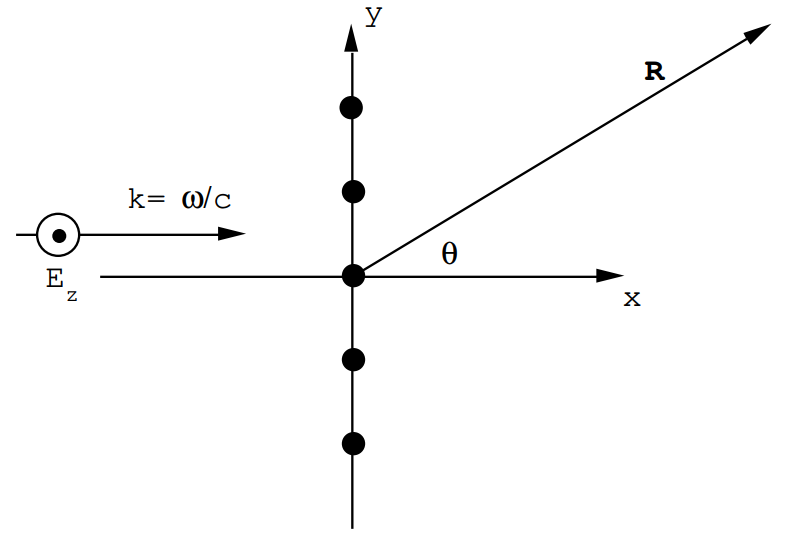A plane wave whose electric field is given by

$\text{E}_{\text{z}}=\text{E}_{\text{O}} \text{e}^{\text{i}(\text{kx}-\omega \text{t})}\nonumber$

is incident upon 5 hydrogen atoms which are spaced a distance a = 1.5 x 10-10 meters along the y-axis as shown in the sketch. The frequency associated with the electric field is 1018 Hz.

An observer in the x-y plane is located very far away in a position specified by the angle θ shown in the sketch.

(a) Calculate the structure factor for the scattered radiation.

(b) Make a sketch of the angular variation of the intensity measured by P as θ ranges from 0 to $$\pi$$/2.

Answer (9.9).

The electric field amplitude at R due to a single atom is independent of θ and the electric field is polarized along z. However, the fields from the 5 atoms interfere because for fixed time of observation, the phase of each wave is shifted. The structure factor is given by

$S=1+e^{-i q \cdot r}_{1}+e^{-i q \cdot r}_{2}+---+e^{-i q \cdot r_{5}}\nonumber$

where $$\mathbf{q}=\left(\mathbf{k}_{\text{f}}-\mathbf{k}_{\text{i}}\right)$$.

In this problem

$$\begin{array}{l} \mathbf{k}_{\text{i}}=\frac{\omega}{\text{c}} \hat{\mathbf{u}}_{\text{x}} \\ \mathbf{k}_{\text{f}}=\frac{\omega}{\text{c}}\left[\cos \theta \hat{\mathbf{u}}_{\text{x}}+\sin \theta \hat{\mathbf{u}}_{\text{y}}\right] \end{array}$$

$$\therefore \text{q}=\frac{\omega}{\text{c}}\left[(\cos \theta-1) \hat{\text{u}}_{\text{x}}+\sin \theta \hat{\text{u}}_{\text{y}}\right]$$.

The atomic positions are given by

$\mathbf{r}_{n}=n \text { a } \hat{\mathbf{u}}_{y}\nonumber$

$\therefore \quad \mathbf{q} \cdot \mathbf{r}_{n}=n \frac{a \omega}{c} \sin \theta=n \phi.\nonumber$

Thus $$S=1+e^{-i \phi}+e^{-2 i \phi}+e^{i \phi}+e^{2 i \phi}$$

(the first term corresponds to n = 0),

or $$S=1+2 \cos \phi+2 \cos 2 \phi$$,

or $$S=1+2 \cos \left(\frac{a \omega}{c} \sin \theta\right)+2 \cos \left(\frac{2 a \omega}{c} \sin \theta\right)$$

In this problem $$\frac{\omega}{c}=\frac{2 \pi}{3} \times 10^{10}$$ and $$a=\frac{3}{2} \times 10^{-10} \text{m}$$.

$$\therefore \frac{a \omega}{c}=\pi$$ and

$$S=1+2 \cos (\pi \sin \theta)+2 \cos (2 \pi \sin \theta)$$

(See the figure below).The intensity measured at P is proportional to |S|2, or to S2 in this case since S is real. Note the strong forward scattering pattern (see the figure below).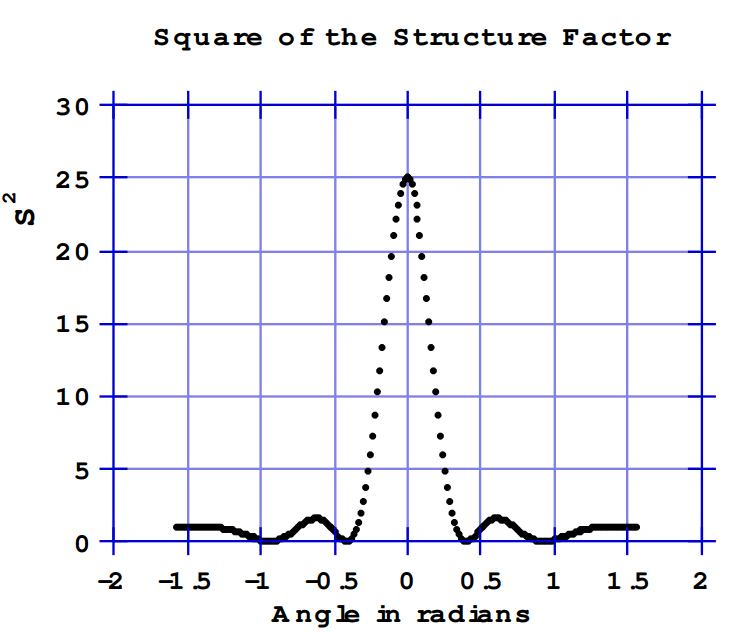Problem (9.10).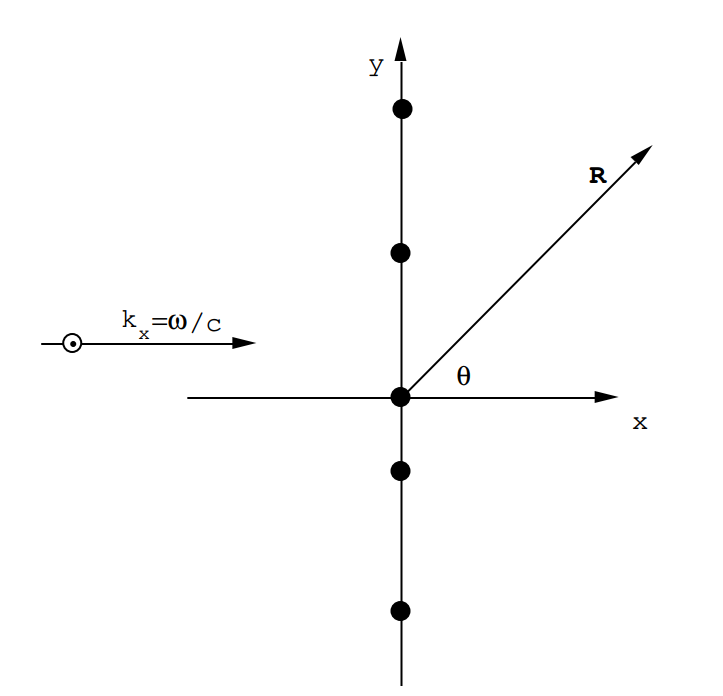This is a repeat of the previous problem but the scattering centers are not equally spaced. A plane wave is incident from the left $$\text{E}_{\text{z}}=\text{E}_{\text{O}} \text{e}^{\text{i}(\text{kx}-\omega \text{t})}$$

The atoms are at:

$$\begin{array}{ll} \mathbf{r}_{1}=0 & \\ \mathbf{r}_{2}=\frac{8 a}{7} \hat{\mathbf{u}}_{\text{y}} & \mathbf{r}_{3}=\left(\frac{16 \text{a}}{7}\right) \hat{\mathbf{u}}_{\text{y}} \\ \mathbf{r}_{4}=-\left(\frac{4 \text{a}}{7}\right) \hat{\mathbf{u}}_{\text{y}} & \mathbf{r}_{5}=-\left(\frac{12 \text{a}}{7}\right) \hat{\mathbf{u}}_{\text{y}}. \end{array}$$

This is a more or less random spacing which preserves an average spacing of a.

Calculate the dependence upon the angle θ of the intensity of the scattered radiation which would be observed at a distant point P. a = 1.5 x 10-10 m and ω = 2$$\pi$$ x 1018 rad./sec.

Answer (9.10).

$$\mathbf{k}_{i}=\frac{\omega}{\text{c}} \hat{\mathbf{u}}_{\text{x}}$$

$$\mathbf{k}_{\text{f}}=\frac{\omega}{\text{c}}\left[\cos \theta \hat{\mathbf{u}}_{\text{x}}+\sin \theta \hat{\mathbf{u}}_{\text{Y}}\right]$$

$$\therefore \mathbf{q}=\left(\mathbf{k}_{\text{f}}-\mathbf{k}_{\text{i}}\right)=\frac{\omega}{\text{C}}\left[(\cos \theta-1) \hat{\mathbf{u}}_{\text{x}}+\sin \theta \hat{\mathbf{u}}_{\text{y}}\right]$$

$$\therefore \mathbf{q} \cdot \mathbf{r}_{1}=0 \quad\quad \quad \mathbf{q} \cdot \mathbf{r}_{2}=\frac{8}{7}\left(\frac{a \omega}{c}\right) \quad \sin \theta$$

$$\mathbf{q} \cdot \mathbf{r}_{3}=\left(\frac{16}{7}\right) \left(\frac{a \omega}{c}\right) \sin \theta$$

$$\mathbf{q} \cdot \mathbf{r}_{4}=-\left(\frac{4}{7}\right)\left(\frac{a \omega}{c}\right) \sin \theta$$

$$\mathbf{q} \cdot \mathbf{r}_{5}=-\left(\frac{12}{7}\right)\left(\frac{\text{a} \omega}{\text{c}}\right) \sin \theta$$

$$\frac{\omega}{c}=\left(\frac{2 \pi}{3}\right)\left(10^{10}\right) \quad \quad \quad \quad a=\frac{3}{2} \times 10^{-10} \quad \quad \quad \therefore \frac{a \omega}{c}=\pi$$

$$S=1+e^{-i} 8 \pi \sin \theta / 7+e^{-i} 16 \pi \sin \theta / 7+e^{i} 4 \pi \sin \theta / 7+e^{i} 12 \pi \sin \theta / 7$$

$$S=\left[1+\cos \left[\left(\frac{8 \pi}{7}\right) \sin \theta\right]+\cos \left[\left(\frac{16 \pi}{7}\right) \sin \theta\right]+\cos \left[\left(\frac{4 \pi}{7}\right) \sin \theta\right]\right.\left.+\cos \left[\left(\frac{12 \pi}{7}\right) \sin \theta\right)\right]$$

$$+i \left[-\sin \left[\left(\frac{8 \pi}{7}\right) \sin \theta\right]-\sin \left[\left(\frac{16 \pi}{7}\right) \sin \theta\right]+\sin \left[\frac{4 \pi}{7} \sin \theta\right]\right.+\sin \left[\left(\frac{12 \pi}{7}\right) \sin \theta\right]$$

The structure factor can be written S = a + ib, then the intensity required is proportional to

|S|2 = SS* = a2 + b2

The result of the calculation is shown in the figures. The main peak at θ = 0 persists because all signals remain in phase no matter where the scatterers are located along the y axis. The main effect of the irregular spacing is to reduce the structure in the "wings" i.e. the oscillations at angles larger than 30˚.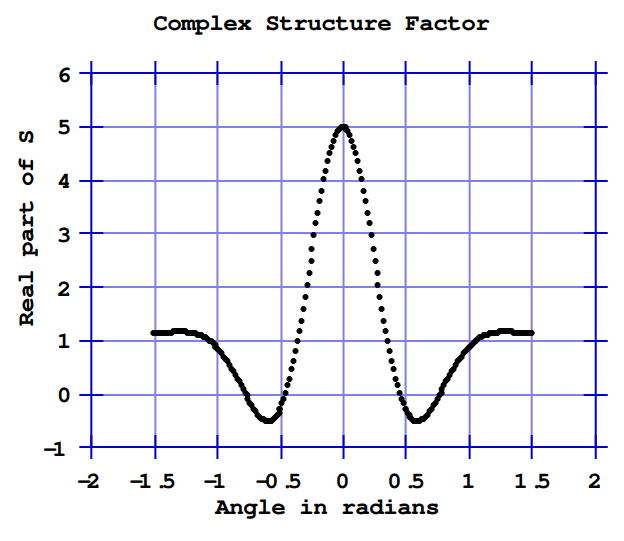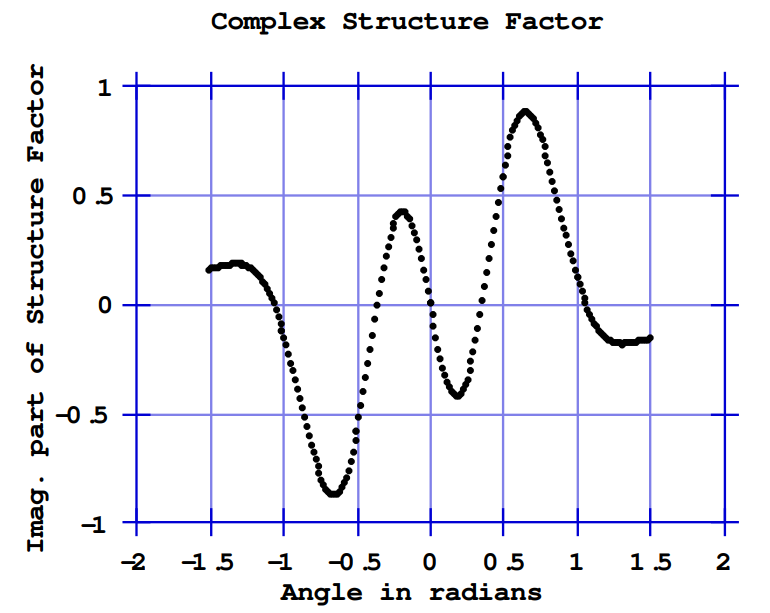Problem (9.11).Four scattering centers are arranged on the grid shown above. A plane wave is incident from the left:

$$\text{E}_{\text{z}}=\text{E}_{\text{O}} \text{e}^{\text{i}(\text{kx}-\omega \text{t})}$$

where ω = 2$$\pi$$ x 1018 rad/sec. The parameter $$a=\frac{3}{2} \times 10^{-10} \text{m}$$ and $$\frac{a \omega}{c}=\pi$$. Calculate the dependence of the scattered intensity on the angle of observation θ when the observer is very far away (R >> a).

Answer (9.11).

The structure factor is given by $$S=\sum_{n} \exp \left(-i \mathbf{q} \cdot \mathbf{r}_{n}\right)$$

where q = kf - ki

In this case $$\mathbf{q}=\frac{\omega}{c}\left[(\cos \theta-1) \hat{\mathbf{u}}_{x}+\sin \theta \hat{\mathbf{u}}_{y}\right]$$

$$\therefore S=e^{-i \frac{\omega a}{C}}[(\cos \theta-1)+\sin \theta]+e^{-i \frac{\omega a}{c}}[(\cos \theta-1)-\sin \theta]+e^{-i \frac{\omega a}{a}}[-(\cos \theta-1)+\sin \theta]+e^{+i \frac{\omega a}{c}}[(\cos \theta-1)+\sin \theta]$$

or for $$\frac{a \omega}{c}=\pi$$

$$S=2 \cos \pi[\cos \theta+\sin \theta-1]+2 \cos \pi[\cos \theta-\sin \theta-1]$$.

This is real because of the symmetry around the origin. S2 is plotted in the figure below.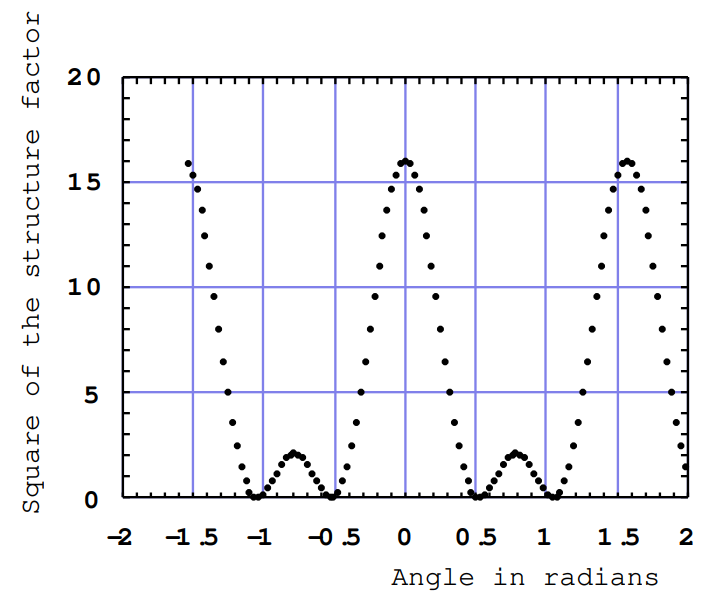• Was this article helpful?